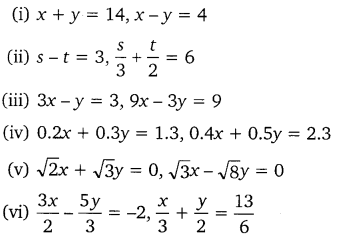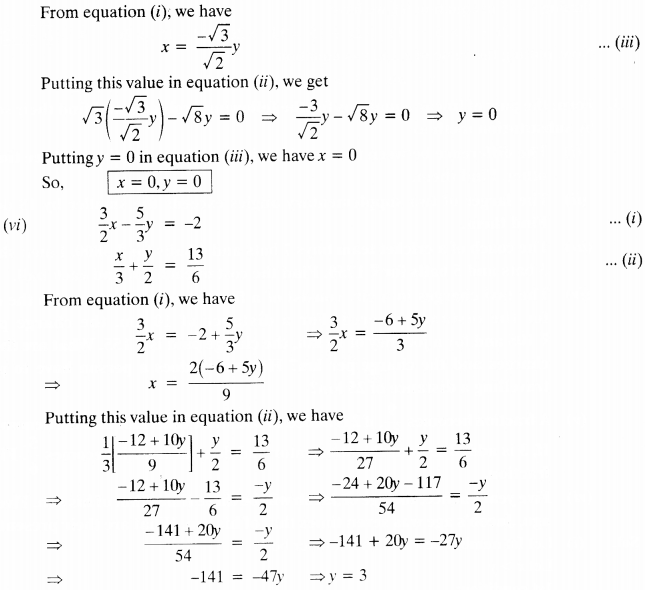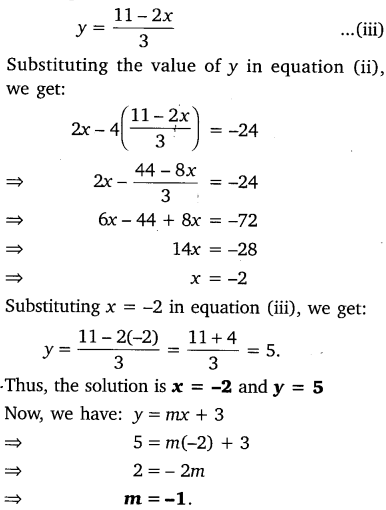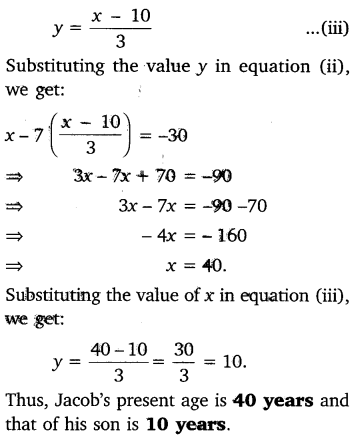# CLASS 10 MATH NCERT SOLUTIONS FOR CHAPTER – 3 PAIR OF LINEAR EQUATIONS IN TWO VARIABLES EX – 3.3

## Pair of Linear Equations in Two Variables

Question 1.
Solve the following pairs of linear equations by the substitution method:Solution:

(i) x + y = 14 …..(i)
x – y = 4   ……(ii)
From equation (ii), we have:
y = x – 4 ….(iii)
Substituting the value of y in equation (i), we get:
x + x-4 =14
⇒   2x = 18
⇒ x = 9
Substituting x = 9 in equation (iii), we get: y = 9 – 4 = 5
Thus, the solution is x = 9 and y = 5.∴ y can have infinite real values
∴ x can have infinite real values because x =$\frac { y+3 }{ 3 }$Question 2.
Solve 2x + 3y = 11 and 2x – 4y = -24 and hence find the value of’m’ for which y = mx +3.

Solution:
2x + 3y = 11  …(i)
2x – 4y = -24 …(ii)
From equation (i), we have:Question 3.
Form the pair of linear equations for the following problems and find their solution by substitution method:

(i) The difference between two numbers is 26 and one number is three times the other. Find them.

(ii) The larger of two supplementary angles exceeds the smaller by 18 degrees. Find them.

(iii) The coach of a cricket team buys 7 bats and 6 balls for ₹3800. Later, she buys 3 bats and 5 balls for ₹1750. Find the cost of each bat and each ball.

(iv) The taxi charges in a city consist of a fixed charge together with the charge for the distance covered. For a distance of 10 km, the charge paid is ₹105 and for a journey of 15 km, the charge paid is ₹155. What are the fixed charges and the charges per km? How much does a person have to pay for travelling a distance of 25 km?

(v) A fraction becomes$\frac { 9 }{ 11 }$, if 2 is added to both the numerator and the denominator. If 3 is added to both the numerator and the denominator, it becomes$\frac { 5 }{ 6 }$. Find the fraction.

(vi) Five years hence, the age of Jacob will be three times that of his son. Five year ago, Jacob’s age was seven times that of his son. What are their present ages?

Solution:

(i) Let the one number be x and the other number be y.
Then according to the question:
x -y = 26    …(i)
and x = 3y    ..(ii)
Substituting the value of x from equation in equation (i), we get:
3y – y = 26
⇒  2y = 26
⇒  y = 13.
Substituting the value of y in equation (ii), we get:
x = 3 x 13 = 39.
Thus, one number is 39 and the other is 13.

(ii) Let the supplementary angles be x and y.
Then according to the question, we have:
x + y = 180°      …(i)
and    x – y = 18°  …(ii)
From equation (ii), we have:
y = x – 18° …(iii)
Substituting the value of y in equation (i), we get:
x + x – 18 = 180°
⇒  2x = 180° + 18° = 198°
⇒  x = 99°.
Substituting the value of x in equation (iii), we get:
y = 990 – 18° = 81°.
Thus, the measures of supplementary angles are 99° and 81°.

(iii) Let the cost of 1 bat and 1 ball be ₹x and ₹y respectively.
Then according to the question, we have:
7x + 6y = 3800           …(i)
and 3x + 5y = 1750   …(ii)
From equation (ii), we have(iv) Let the fixed charges be ₹x and subsequent charges per km be ₹y.
Then according to the question, we have:
x + 10y = 105  …(i)
and x + 15y = 155  …(ii)
From equation (i), we have:(v) Let the fraction be$\frac { x }{ y }$.
Then according to the first condition, we have:(vi) Let the present age of Jacob be x years and that of his son be y years.
Then five years after, their ages will be (x + 5) and (y + 5) respectively.
According to the first condition, we have:
(x + 5) = 3(y + 5)
⇒ x + 5 = 3y + 15
⇒ x – 3y = 10 …..(i)
Five years ago, their ages were (x – 5) and (y – 5) respectively.
According to the second condition, we have: